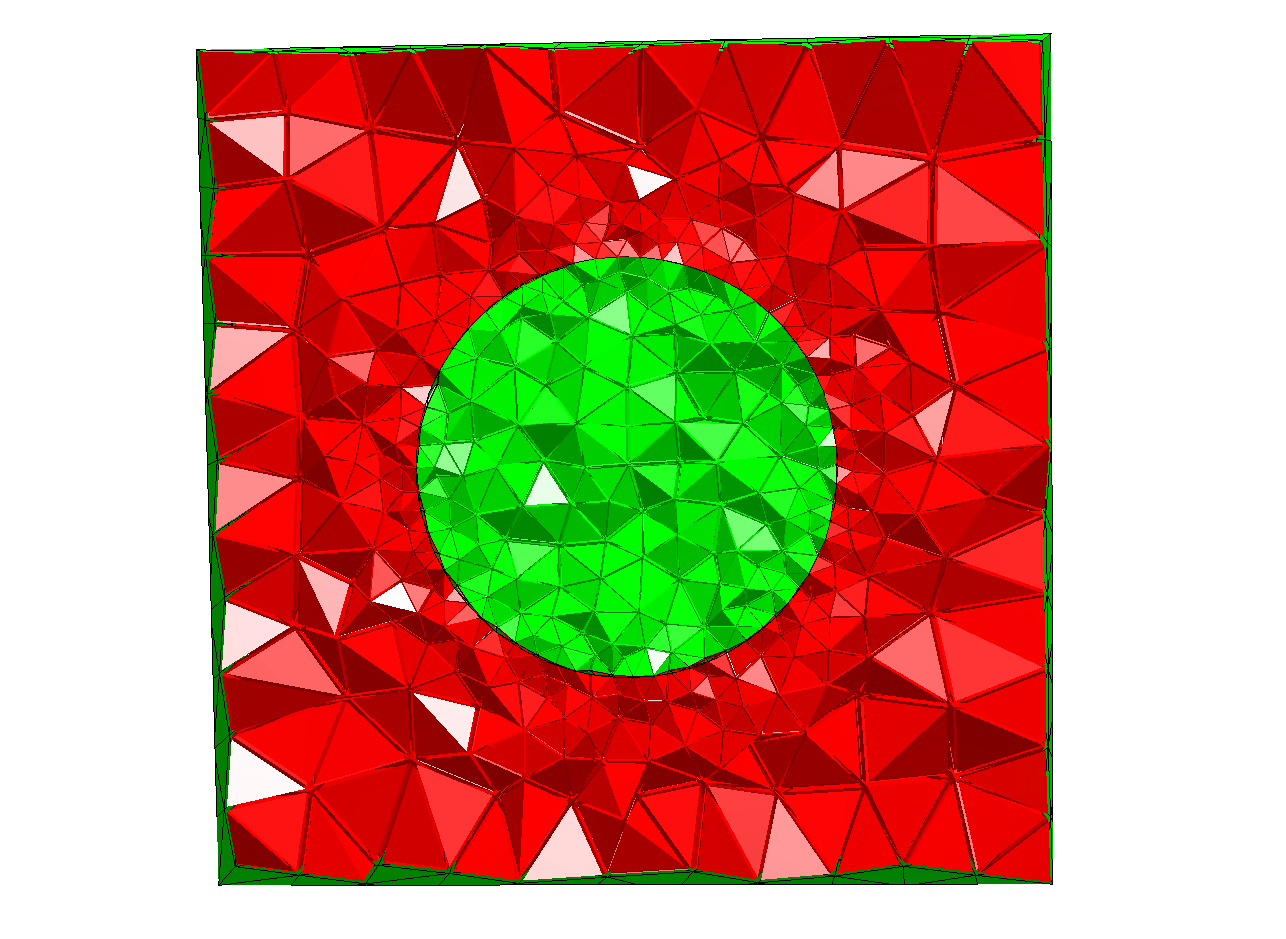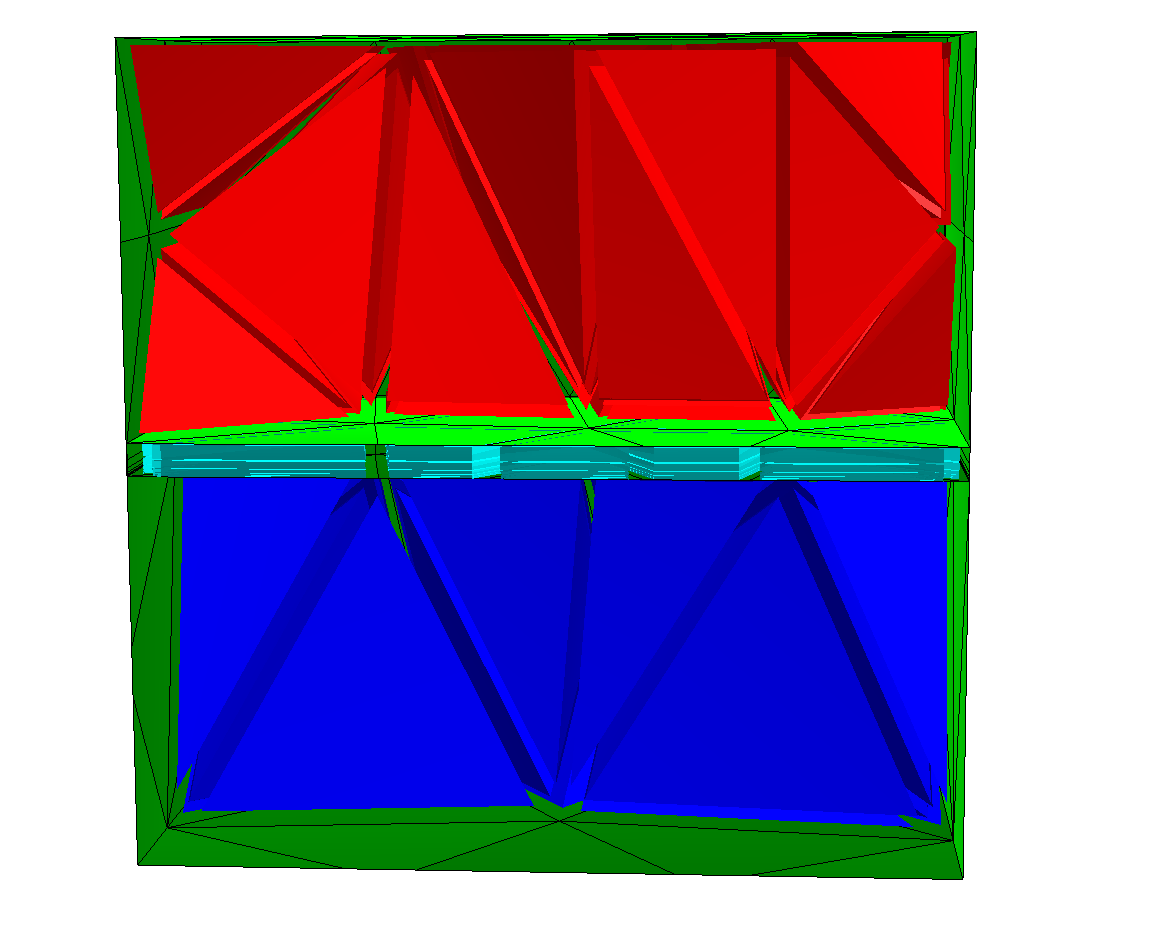# Mesh Size and Refinement¶

## CSGeometry¶

### Local Mesh Size¶

Local mesh sizes of boundaries are given to the solid object itself, you can set the volume mesh size when adding them to the geometry object:

```
from netgen.csg import *

geo = CSGeometry()
# Set the mesh size on the sphere surface to 0.1
sphere = Sphere(Pnt(0,0,0),1).maxh(0.1)
# meshsize of the surface of the brick will not be finer than
# the volume mesh size
brick = OrthoBrick(Pnt(-2,-2,-2),Pnt(2,2,2))
# in the outer region we don't give a local mesh size -> global
# is used
# in the volume of the sphere we set the meshsize to 0.2
# the global mesh size is set to 0.4
ngmesh = geo.GenerateMesh(maxh=0.4)

# for visualization we need a NGSolve mesh
from ngsolve import Mesh
Draw(Mesh(ngmesh))
```### Anisotropic Meshes¶

Netgen can create anisotropic meshes, using the close surface identification. It will fill the layer with prisms, which can be sliced. This is best explained in an example:

```
from netgen.csg import *

geo = CSGeometry()

box = OrthoBrick(Pnt(0,0,0),Pnt(1,1,1))
top = Plane(Pnt(0,0,0.52),Vec(0,0,1))
bot = Plane(Pnt(0,0,0.48),Vec(0,0,-1))
plate = box * top * bot